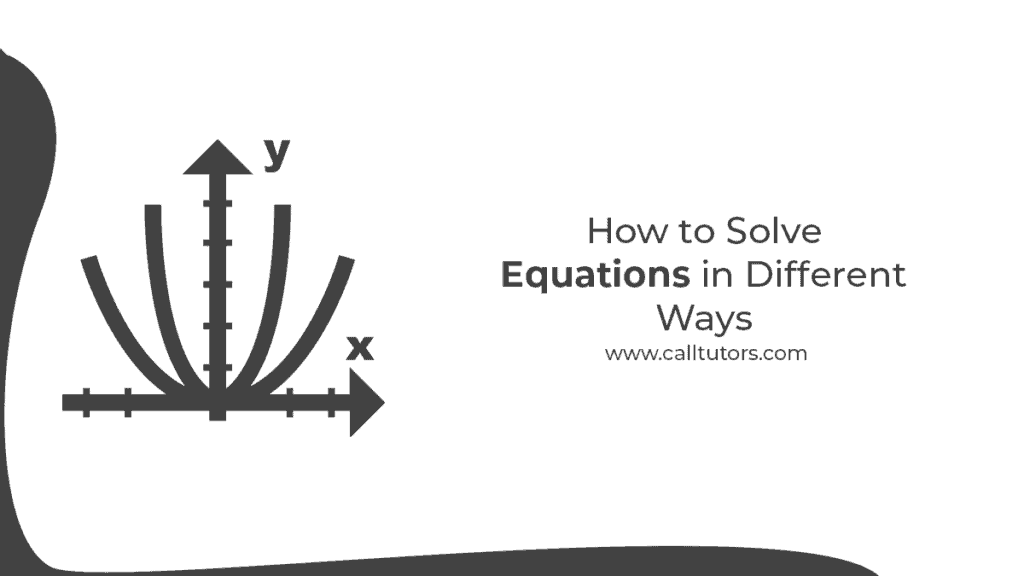# How to Solve Equation in Different Ways

In mathematics, an equation is a type of statement in which two sides or things are equal; this is why equals to sign is used. And this mathematical statement has two sides and the left side is known as the left hand side and the other side is known as the right hand side and these sides are equal to each other. Thus this statement asserts that two expressions are equal. In math, such equations consist of one or more variables like X, Y, Z etc.  We need to find the value of such variables. This is why all the time students ask how to solve equations. But if you know the basics of equations and the properties relating thereto then it becomes much easier to solve such equations.

## Methods – How to solve equation

Summary

### 1.How to solve first degree equations and inequalities –

Let’s learn how to solve first degree equations with the help of an example.

Suppose the equation is “find a number which, when added to 5, yields 10”.

We can write such equation as –

X + 5 = 10.

Now you are required to find the value of X. In this equation,  if you are struggling how to solve the equation then you have to follow the isolation method. So you need to keep the X on the left hand side and then shift the +5 to the other side. When you will take the +5 to the right side then you have to follow the positive properties and according to that it will become -5 on the right side.

X is a variable which you need to find in the equation. This equation is known as the first degree equation as here the power of X is 1 that is the exponent of X is 1.

### 2. Know whether the equation is false or true.

If you are struggling with how to solve the equation then you must know that equation can be true or false. For example the equation is X + 5 = 7. Then if we follow the above method then we will get the value of X as 2. So if you take any other number as the value of X then 2 then the equation will be false as the LHS and RHS will not be equal.

In this equation, X is the variable and we need to find the value of X. and the solution of the equation is 2.

Suppose the equation is 5x – 3 = 4x + 1.

Now you need to get the value of x. For finding the solution of the equation you can put any value in the x then if both the sides are equal it means the value is correct and if the sides are not equal then it means the value of X is incorrect.

Let’s say, you take the value of X as 4 then the equation will be as follows –

5(4) – 3 = 4(4) + 1

17 = 17.

The value of x is true.

### 3. How to solve Equivalent Equations –

If you are struggling with how to solve an equation which has only one solution then you must be stuck with an equivalent equation. When the equation has identical solutions then such equation is called an equivalent equation. It means the equation will have only one solution. For example, the equation is 3x + 3 = 12. For finding the solution we need to isolate the x.  So you have to shift the both the 3s from the left side to the right side. Then we will get the value of x and the value of x shall be x = (12 – 3) / 3 = 3.  This equation has only one solution that is 3. This is why this equation is called an equivalent equation.  In this equation, we have used both addition and subtraction properties to solve the equation.

We can also write such equations in alphabetical forms such as a- b = b – a or a + c = c + a.

### Examples on How to solve equation

Example 1  –

Let’s take an example of an equivalent equation that is X + 2 = 7. And now you have to subtract 2 from each number then you will get the following solution –

X + 2 – 2 = 7 – 2 thus you will get 5 as the solution of x.

If you are stuck with how to solve equations of such nature then you must note that x + 2 = 7 and x = 5 both are equivalent solutions.

Example 2 –

Let’s take another example of an equivalent equation to understand how to solve the equation.

2x -1 – 3x = 5. Now suppose you have to add 2 to both the sides.

2x – 1 – 3x + 2 = 5 + 2

2x – 3x + 1 = 7

2x – 3x = 7 – 1

2x – 3x = 6

-x = 6

X = -6

Thus the value of x is -6.

## Conclusion

Equation is a kind of mathematical statement where you have to find the value of x. This is why many students struggle with how to solve equations. There are different types of equations and which are to be solved accordingly.  If you are also finding ways to solve equations then the article would have helped to understand the basics of equations and what the methods of solving such equations are. Get the best help with math homework from the experts.

This site uses Akismet to reduce spam. Learn how your comment data is processed.